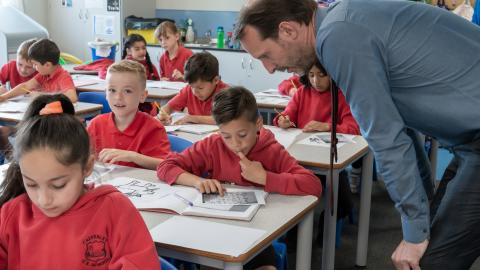## Maths

At Calverley CE we are committed to providing children with an exciting and enriching mathematics curriculum. Children will investigate, problem solve and analyse maths in a wide range of contexts.

The National curriculum for maths has three clear aims:

*Conceptual understanding - Children should become fluent in the fundamentals of maths. With varied practice which increases in complexity over time, pupils should develop a deep understanding and have the ability to recall and apply knowledge rapidly and with accuracy.

*Mathematical reasoning - Children will be able to reason mathematically following a line of enquiry. Through doing this they should be able to test relationships of number and generalisations, and developing an argument, justification or proof using mathematical language.

*Problem solving - Children will solve problems by applying mathematical understanding to a variety of problems. These will gradually increase with sophistication and require the child to break down the problem into a series of simpler steps.

To aid fluency children are given half termly K.I.R.F targets (Key Instant Recall Facts). These targets help to develop essential mental arithmetic skills and can be worked on both at home and in school

Below, you can view the mathematics Long Term Plans (LTP) for each year group.

PDF Attachments